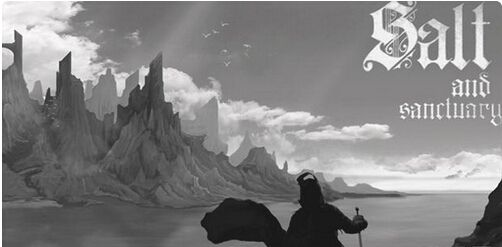# 盐和避难所武器怎么出招,盐和避难所全武器出招操作介绍

《盐和避难所》怎么出招？以下是6399小编为大家带来全武器出招操作介绍。

http://www.v6399.com/game/312/37000/Q=轻击/Z=重击

【通用类大致连招】

Q*N

Z*N

Q+Z=挑飞

【直剑类】

Q+Q+↑Q=第三下跳砍

Q+Q+↓Q=第三下挑飞

Q+Q+Q+Z=3连+击飞

Q+Q+↓Q=第3下挑飞

【剑鞭】

Z+Q=挥鞭+跳砍

【剑类/太刀类】

Q*4算一轮连击，其他通用

【匕首类】

Q+Z=飞踢，然后制空接楼下可连

【副手铳/魔杖】

Q+Z=后跳开枪/施法

【长枪】

Z+↑Q=720°单手螺旋桨

Q+Q↑Q=720°螺旋桨

Q+Q+Z=横向大风车 再+Z可连螺旋

【镰刀】

Q+↑Q=螺旋桨

【装X神器-战斧类】

Q+↑Q=720°螺旋桨

Q+Q+Z=横向大风车

Z+Z+Q=三连最后为Z击

Z+↑Q=720°螺旋桨（实用）